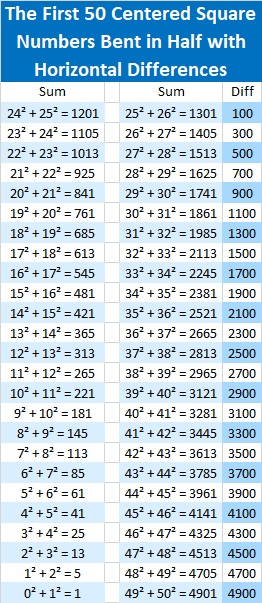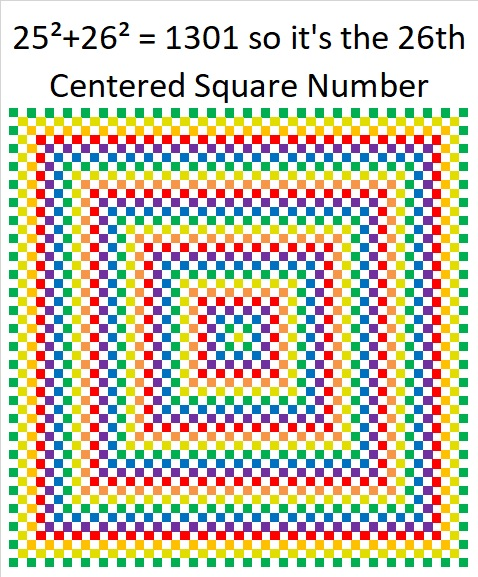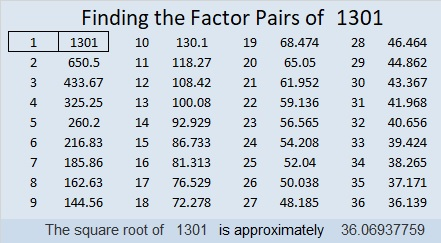# 1301 Cool Patterns of Centered Square Numbers

1301 is a centered square number because it is the sum of two consecutive square numbers. It also happens to be exactly 100 more than the previous centered square number.

1301 is special because it is the sum of two consecutive squares, but it is not the only sum of two squares that is 100 more than another sum of two squares. Here is a chart of some of those squares with some other information. What patterns do you see?What role did the triangular numbers play on that chart? The pattern you see of 4, 12, 24, etc. is not new. Look at this chart of centered square numbers below. Below each centered square number (in blue) are related sums of squares. The difference of those related sums and the centered square above it follows that same 4, 12, 24, . . .  pattern.When you look just at centered square numbers, there is a different pattern for them as well. For example, if you know that 1301 is the 26th centered square number, then you can be sure that 1301 + 4(26) = 1405 will be the next centered square number.When the chart above is “bent in half” so the list of centered square numbers begins at the lower left and ends at the lower right, there is yet another pattern. Look at the differences horizontally to see a cool pattern:I’ve made it clear that centered square numbers are made when consecutive square numbers are added, such as 26² + 25² = 1301, but what does one look like? Here’s a picture of 1301 tiny little squares arranged as a centered square.There are 100 tiny green squares around it because it is 100 more than the previous centered square number.

Here are some more facts about the number 1301:

• 1301 is a prime number and the first prime in a prime triplet.
• Prime factorization: 1301 is prime.
• The exponent of prime number 1301 is 1. Adding 1 to that exponent we get (1 + 1) = 2. Therefore 1301 has exactly 2 factors.
• Factors of 1301: 1, 1301
• Factor pairs: 1301 = 1 × 1301
• 1301 has no square factors that allow its square root to be simplified. √1301 ≈ 36.06938

How do we know that 1301 is a prime number? If 1301 were not a prime number, then it would be divisible by at least one prime number less than or equal to √1301 ≈ 36.1. Since 1301 cannot be divided evenly by 2, 3, 5, 7, 11, 13, 17, 19, 23, 29 or 31, we know that 1301 is a prime number.Every centered square number is a hypotenuse of a Pythagorean triple. For example,
primitive 51-1300-1301 is calculated from 26² – 25², 2(26)(25), 26² + 25²

Here’s another way we know that 1301 is a prime number: Since its last two digits divided by 4 leave a remainder of 1, and 26² + 25² = 1301 with 26 and 25 having no common prime factors, 1301 will be prime unless it is divisible by a prime number Pythagorean triple hypotenuse less than or equal to √1301 ≈ 36.1. Since 1301 is not divisible by 5, 13, 17, or 29, we know that 1301 is a prime number.

This site uses Akismet to reduce spam. Learn how your comment data is processed.